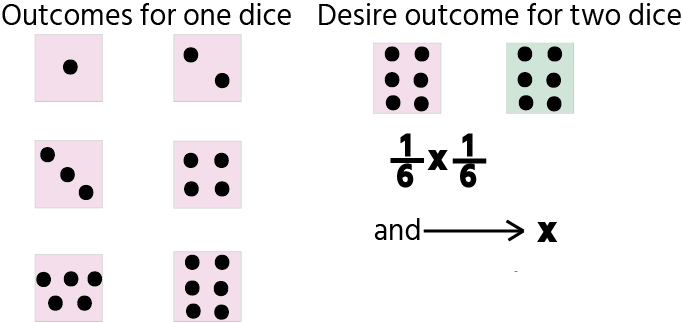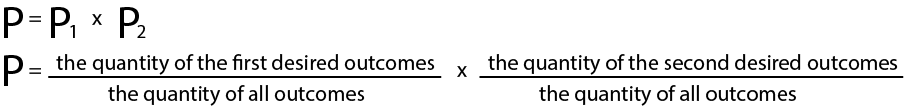Course Content

Probability TheoryMultiply probabilities

Sometimes it is impossible to pick one event, hence, we should refer to the multiplication rule for independent events.

Independent events mean that the occurrence of one event doesn't depend on another event like flipping two coins or rolling two dies.

Let's talk about the multiplication rule.

Where can I use it?

In a situation where we certainly know that we are interested in more than one event.

Example:

Imagine rolling two dice; calculate the probability that the multiplication of numbers on the edge of two dice is `36`. The first one has `6` on its dice and the second one has `6` on its dice.

Explanation:Here is the formula:

Explanation:If we want to receive success for both the first and second events, we should multiply the probability of getting a win in the first event and the second. The key for the multiplication rule is and. If you see the phrase the first <strong data-reactroot="">and</strong> the second event you should use the multiplication rule.#### Calculate the probability that you will succeed in the Math and English test. The probability that you will succeed in Math is 10% or 0.10 and in English is 7% or 0.07. Put items in the needed order. .

Probability = 0.10
___
___

Click or drag`n`drop items and fill in the blanks*0.07+0.7Section 2.

Chapter 4